# Understanding of contact detection UDF

 Register Blogs Members List Search Today's Posts Mark Forums ReadJune 10, 2021, 04:30Understanding of contact detection UDF #1 New Member   Join Date: Feb 2020 Posts: 20 Rep Power: 3Hello, everyone: I am now using ‘contact detection’ function to keep a distance between two faces(one is stationary and the other is moving), some part of the UDF for contact detection is a little hard for me to understand since some macros cannot be found in UDF manual, so I am here asking for help to figure the whole things out. UDF code and my personal understanding of it have been presented in Figure 1 and Figure 2. Any advice will be appreciated, please help me understand those macros and share your point on the UDF code and my corresponding comments, thanks a lot! Regards, Silence UDF macros which cannot be found in UDF manual: 1.What is ‘Objp’? (Part 1, line 6) 2. Loop(o, contacts) (Par2 line 33) Loop the elements which pointer 'o' points to in contacts? 3.face = O_F(o) (Par2 line 35) 4.thread = O_F_THREAD(o) (Par2 line 36) 5.REAL_MIN (Par3 line 79) 6. SDOF_Get_Motion(dt, vel0, omega0, theta0) (Par4 line 113) Get the motion property of the structure which thread dt points to, velocity(vel0), revolution speed(omega0) and degree produced by revolution(theta0), am I right? 7. SDOF_Overwrite_Motion(ndt, vel1, omega1, theta1) (Par5 line 144) Overwrite the motion property of thread ‘ndt’, the new property of motion is: velocity_new = vel1, revolution speed_new = omega1 and degree_new = theta1, am I right? Logic problem 1./* 'Fetch current thread ID'---UDF manual, */, what does 'current thread' mean? (part 2, line 29), 2.tid = THREAD_ID(DT_THREAD(dt)), 'dt' was not defined in previous statement, why it can be used here? (part 2, line 30) 3.nid = -1; what is nid? why nid = -1? (part 2, line 31) 4.n_faces++, Obtain the total number of faces on face ‘nid’? (part 2, line 56) 5. N3V_S(xc, /=, n_faces), Is this operation to obtain the centroid of whole face? if so, is it an axiom? (part, line 81) 6. ndt = THREAD_DT(thread), convert thread to dynamic thread, thread is the pointer of face ‘nid’, why do this operation? (part 4, line 110) 7./* Reflect velocity across the normal */ v1dotn0 = 2 * N3V_DOT(vel1, norm0); N3V_S(norm0, *=, v1dotn0); N3V_V(vel1, -=, norm0); why not just N3V_V(vel1, -=, 2*vel1), why obtain the final vel1 through normal vector? (part 5, line 139-142)?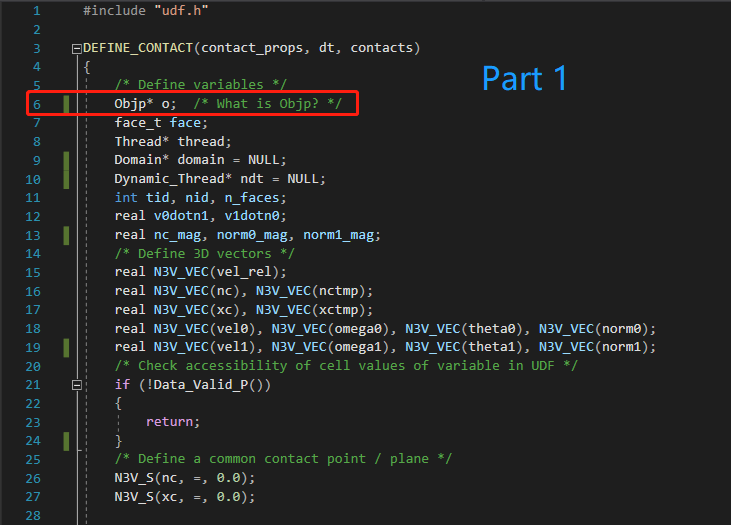Figure 1a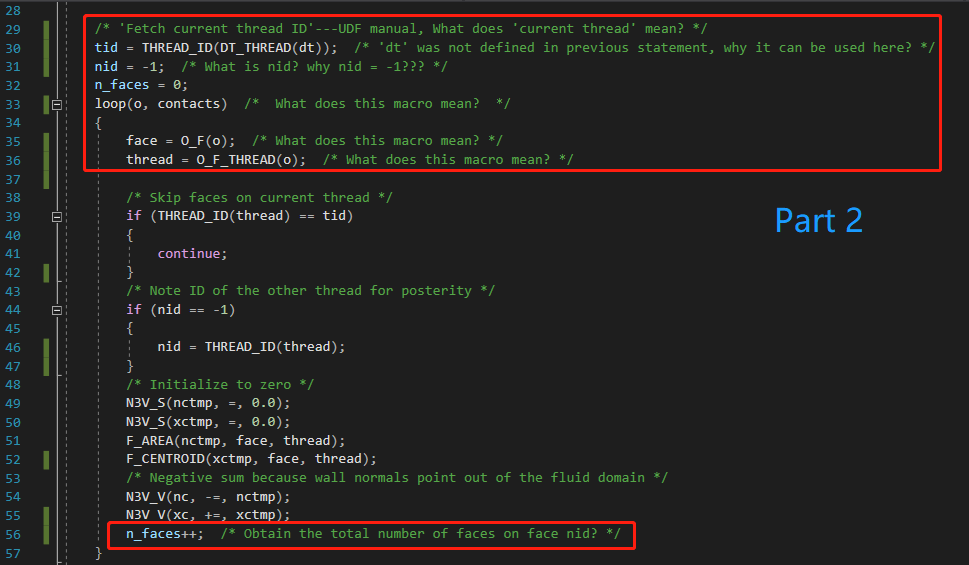Figure 1b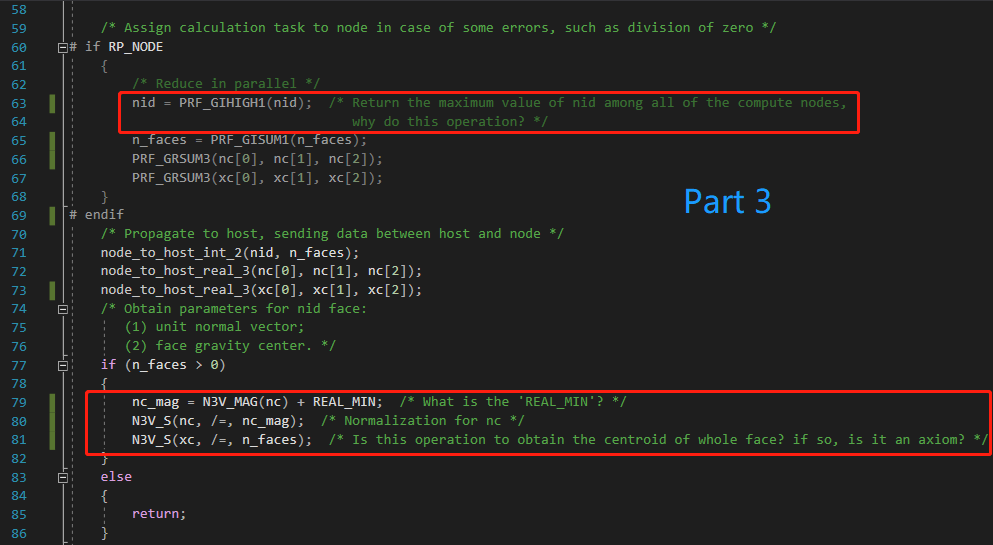Figure 1c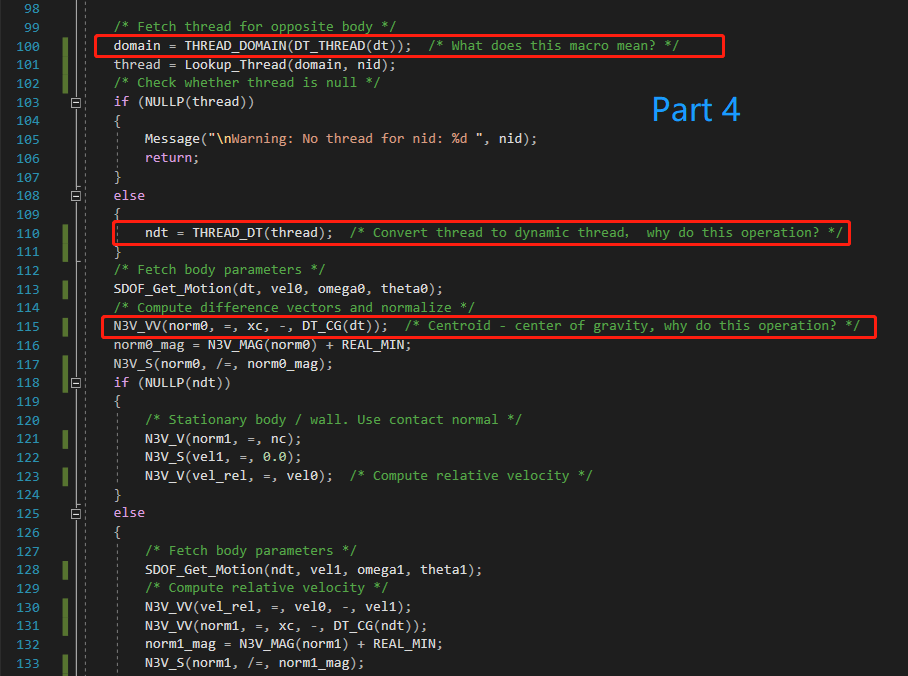Figure 1d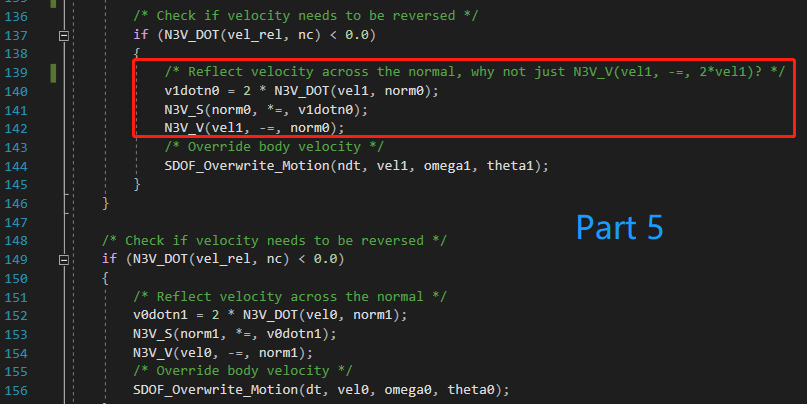Figure 1e Code: ```#include "udf.h" DEFINE_CONTACT(contact_props, dt, contacts) { /* Define variables */ Objp* o; /* What is Objp? */ face_t face; Thread* thread; Domain* domain = NULL; Dynamic_Thread* ndt = NULL; int tid, nid, n_faces; real v0dotn1, v1dotn0; real nc_mag, norm0_mag, norm1_mag; /* Define 3D vectors */ real N3V_VEC(vel_rel); real N3V_VEC(nc), N3V_VEC(nctmp); real N3V_VEC(xc), N3V_VEC(xctmp); real N3V_VEC(vel0), N3V_VEC(omega0), N3V_VEC(theta0), N3V_VEC(norm0); real N3V_VEC(vel1), N3V_VEC(omega1), N3V_VEC(theta1), N3V_VEC(norm1); /* Check accessibility of cell values of variable in UDF */ if (!Data_Valid_P()) { return; } /* Define a common contact point / plane */ N3V_S(nc, =, 0.0); N3V_S(xc, =, 0.0); /* 'Fetch current thread ID'---UDF manual, What does 'current thread' mean? */ tid = THREAD_ID(DT_THREAD(dt)); /* 'dt' was not defined in previous statement, why it can be used here? */ nid = -1; /* What is nid? why nid = -1??? */ n_faces = 0; loop(o, contacts) /* What does this macro mean? */ { face = O_F(o); /* What does this macro mean? */ thread = O_F_THREAD(o); /* What does this macro mean? */ /* Skip faces on current thread */ if (THREAD_ID(thread) == tid) { continue; } /* Note ID of the other thread for posterity */ if (nid == -1) { nid = THREAD_ID(thread); } /* Initialize to zero */ N3V_S(nctmp, =, 0.0); N3V_S(xctmp, =, 0.0); F_AREA(nctmp, face, thread); F_CENTROID(xctmp, face, thread); /* Negative sum because wall normals point out of the fluid domain */ N3V_V(nc, -=, nctmp); N3V_V(xc, +=, xctmp); n_faces++; /* Obtain the total number of faces on face nid? */ } /* Assign calculation task to node in case of some errors, such as division of zero */ # if RP_NODE { /* Reduce in parallel */ nid = PRF_GIHIGH1(nid); /* Return the maximum value of nid among all of the compute nodes, why do this operation? */ n_faces = PRF_GISUM1(n_faces); PRF_GRSUM3(nc, nc, nc); PRF_GRSUM3(xc, xc, xc); } # endif /* Propagate to host, sending data between host and node */ node_to_host_int_2(nid, n_faces); node_to_host_real_3(nc, nc, nc); node_to_host_real_3(xc, xc, xc); /* Obtain parameters for nid face: (1) unit normal vector; (2) face gravity center. */ if (n_faces > 0) { nc_mag = N3V_MAG(nc) + REAL_MIN; /* What is the 'REAL_MIN'? */ N3V_S(nc, /=, nc_mag); /* Normalization for nc */ N3V_S(xc, /=, n_faces); /* Is this operation to obtain the centroid of whole face? if so, is it an axiom? */ } else { return; } #if RP_HOST Message ( "\nContact:: tid: %d nid: %d n_faces: %d " "Point: (%f %f %f) Normal: (%f %f %f)", tid, nid, n_faces, xc, xc, xc, nc, nc, nc ); #endif /* Fetch thread for opposite body */ domain = THREAD_DOMAIN(DT_THREAD(dt)); /* What does this macro mean? */ thread = Lookup_Thread(domain, nid); /* Check whether thread is null */ if (NULLP(thread)) { Message("\nWarning: No thread for nid: %d ", nid); return; } else { ndt = THREAD_DT(thread); /* Convert thread to dynamic thread， why do this operation? */ } /* Fetch body parameters */ SDOF_Get_Motion(dt, vel0, omega0, theta0); /* Compute difference vectors and normalize */ N3V_VV(norm0, =, xc, -, DT_CG(dt)); /* Centroid - center of gravity, why do this operation? */ norm0_mag = N3V_MAG(norm0) + REAL_MIN; N3V_S(norm0, /=, norm0_mag); if (NULLP(ndt)) { /* Stationary body / wall. Use contact normal */ N3V_V(norm1, =, nc); N3V_S(vel1, =, 0.0); N3V_V(vel_rel, =, vel0); /* Compute relative velocity */ } else { /* Fetch body parameters */ SDOF_Get_Motion(ndt, vel1, omega1, theta1); /* Compute relative velocity */ N3V_VV(vel_rel, =, vel0, -, vel1); N3V_VV(norm1, =, xc, -, DT_CG(ndt)); norm1_mag = N3V_MAG(norm1) + REAL_MIN; N3V_S(norm1, /=, norm1_mag); /* Check if velocity needs to be reversed */ if (N3V_DOT(vel_rel, nc) < 0.0) { /* Reflect velocity across the normal, why not just N3V_V(vel1, -=, 2*vel1)? */ v1dotn0 = 2 * N3V_DOT(vel1, norm0); N3V_S(norm0, *=, v1dotn0); N3V_V(vel1, -=, norm0); /* Override body velocity */ SDOF_Overwrite_Motion(ndt, vel1, omega1, theta1); } } /* Check if velocity needs to be reversed */ if (N3V_DOT(vel_rel, nc) < 0.0) { /* Reflect velocity across the normal */ v0dotn1 = 2 * N3V_DOT(vel0, norm1); N3V_S(norm1, *=, v0dotn1); N3V_V(vel0, -=, norm1); /* Override body velocity */ SDOF_Overwrite_Motion(dt, vel0, omega0, theta0); } #if RP_HOST Message ( "\ncontact_props: Updated :: vel0 = (%f %f %f) vel1 = (%f %f %f)", vel0, vel0, vel0, vel1, vel1, vel1 ); #endif }```Tags contact detection, udf codeThread Tools Search this ThreadShow Printable VersionEmail this Page Search this Thread: Advanced Search Display ModesLinear ModeSwitch to Hybrid ModeSwitch to Threaded ModePosting Rules You may not post new threads You may not post replies You may not post attachments You may not edit your posts BB code is On Smilies are On [IMG] code is On HTML code is OffTrackbacks are Off Pingbacks are On Refbacks are On Forum RulesSimilar Threads Thread Thread Starter Forum Replies Last Post Wolfgang.Black FLOW-3D 0 May 26, 2020 15:20 pchoopanya Fluent UDF and Scheme Programming 1 August 28, 2013 06:12 gandesk Fluent UDF and Scheme Programming 1 November 1, 2010 09:00 smkhan Main CFD Forum 1 July 10, 2010 08:24 Aireen FLUENT 0 August 11, 2006 11:08

All times are GMT -4. The time now is 23:11.